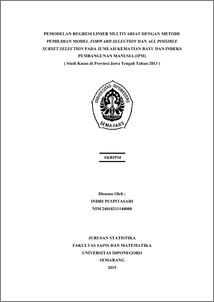# PEMODELAN REGRESI LINIER MULTIVARIAT DENGAN METODE PEMILIHAN MODEL FORWARD SELECTION DAN ALL POSSIBLE SUBSET SELECTION PADA JUMLAH KEMATIAN BAYI DAN INDEKS PEMBANGUNAN MANUSIA (IPM) ( Studi Kasus di Provinsi Jawa Tengah Tahun 2013 )

PUSPITASARI, INDRI (2015) PEMODELAN REGRESI LINIER MULTIVARIAT DENGAN METODE PEMILIHAN MODEL FORWARD SELECTION DAN ALL POSSIBLE SUBSET SELECTION PADA JUMLAH KEMATIAN BAYI DAN INDEKS PEMBANGUNAN MANUSIA (IPM) ( Studi Kasus di Provinsi Jawa Tengah Tahun 2013 ). Undergraduate thesis, FSM Universitas Diponegoro.Preview
PDF
3345Kb

## Abstract

Regression analysis is a statistical analysis that aims to measure the effect of the independent variables to the dependent variable. Multivariate Linear Regression is a regression model that consists of more than one dependent variables and the dependent variables are correlated. The Number of Infant Mortality and Human Development Index (HDI) of Central Java Province in 2013 was influenced by several variables, such as: mean years of schooling and the number of health centers. To analyze the effects of mean years of schooling and the number of health centers to The Number of Infant Mortality and Human Development Index (HDI) can use multivariate linear regression analysis becuase the dependent variables are correlated. Model selection is determined by using the Forward Selection and All Possible Subset Selection. Selection the model by using Forward Selection, first variables that is included in the model is based of independent variable that have the greatest correlation with the dependent variables. For All Possible Subset Selection, model selection is done by modeling all the models that may have formed. AIC criteria is used for determining the model for All Possible Subset Selection. The model which is selected by using Forward Selection and All Possible Subset Selection has the same independent variables, the model with independent variables mean years of schooling and the number of health centers. The error of the model fulfill all the assumptions of error. Based on the model, the value of AIC is 247.8142 and Eta Squared Lambda is 92.22%. Keywords : Multivariate Linear Regression, Forward Selection, All Possible Subset Selection, AIC, IPM, Number of Infant Mortality, Central Java.

Item Type: Thesis (Undergraduate) H Social Sciences > HA Statistics Faculty of Science and Mathematics > Department of Statistics 47304 Mr Hasbi Yasin 05 Jan 2016 17:37 05 Jan 2016 17:37

Repository Staff Only: item control page